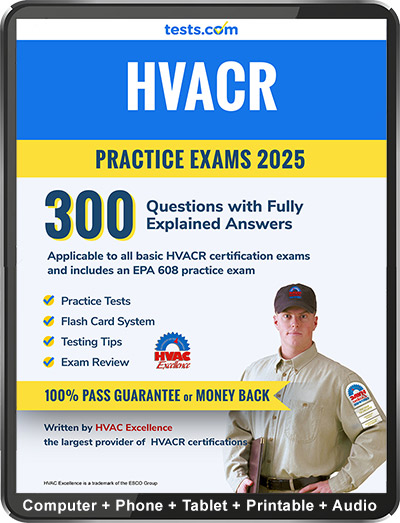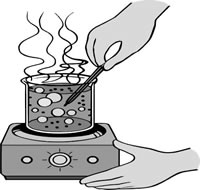2020 Edition

# HVAC Practice Test

HVAC practice exams can be used for professional-growth and to prepare for certification exams. Our HVACR practice exam consists of questions developed by HVAC Excellence, the largest provider of certifications (more than 200,000) in the HVACR industry.

• assessment of broad based HVACR knowledge
• practice in the various core specialty areas
• benefit for self-assessment and professional-growth, as well as for exam preparation
• use for students in trade-schools studying for course exams and for professional technicians sitting for certification exams
• a good opportunity for practicing test taking skills and learning the subject matter

A 100 question EPA 608 practice test comes with our materials.

Take this free HVAC practice test to see how prepared you are for an HVAC certification exam.

 1. Two locations where a cold air return should be installed: a. Open area of wall and low to the ground. b. Behind appliances and high on the wall. c. Open area of wall and high on the wall. d. Behind appliances and low to the ground.
 2. Which of the following is a law of thermodynamics: a. Heat is a form of matter. b. Heat moves toward a place with higher intensity. c. Heat moves toward a place with lower intensity. d. Heat moves toward a place with a higher temperature.
 3. Sensible heat describes _________________________. a. How fast heat will travel. b. The quantity of heat. c. The volume of heat. d. How hot something feels.
 4. Latent heat measures _______________________. a. The temperature of heat in a substance. b. The quantity of heat in a substance. c. The velocity of heat in a substance. d. The heat potential of a substance.
 5. Latent heat is measured in ____________________. a. Degrees Celsius, F ahrenheit and Kelvin b. International System of Units c. British Thermal Units d. Board of Trade Unit
 6. If 1 pound of water warms to 60 degrees F from 55 degrees F, what btu of latent heat will it have absorbed? a. 2.5 b. 5 c. 10 d. 15
Study Online InstantlyClick to Save 50% Now
 7. What is the amount of heat energy required to evaporate 1 pound of water? a. 370 btu b. 570 btu c. 770 btu d. 970 btu
 8. In an air conditioning and refrigeration system, what occurs in an evaporator? a. The refrigerant absorbs the latent heat. b. The refrigerant evaporates latent heat. c. Latent heat is condensed. d. Latent heat is released.
 9. In an air conditioning and refrigeration system, what occurs in a condenser? a. The refrigerant absorbs the latent heat. b. The refrigerant releases the latent heat. c. Latent heat is pressurized. d. Latent heat is increased.
 10. In a sealed system, pressure and temperature _________________. a. are inversely proportional b. go in opposite directions up and down c. are equal d. follow each other up and down
 11. Which of the following is not a type of compressor? a. Lateral b. Reciprocating c. Rotary d. Screw e. Centrifugal
 12. In Fahrenheit, the boiling point of water is _____________. a. 100 degrees b. 112 degrees c. 212 degrees d. 221 degrees
 13. To change Fahrenheit to Celsius, which formula is used?a. C =(F+32)÷ 1.8 b. C=(F-32) x 1.8 c. C=(F-32) ÷ 1.8 d. C= (F-32)+1.8
 14. Which of the following is not a method by which heat may be transferred from a warmer substance to a colder substance? a. Conduction b. Retraction c. Convection d. Radiation
 15. What btu of heat is required to raise 1 pound of ice 1 degree F when the temperature is below 32 degrees F? a. .25 b. .5 c. 1 d. 1.5
 16. What btu of heat is required to raise 1 pound of steam 1 degree F above the temperature of 212 degrees F? a. .25 b. .5 c. 1 d. 1.5
 17. A day-ton of refrigeration is the amount of refrigeration produced by melting 1 ton of ice at a temperature of 32 degrees F in 24 hours. True False
 18. Ice exerts pressure ________________. a. Upwards b. Laterally c. Downwards d. In all directions
 19. Pressure is usually measured in ___________. a. Pounds per square foot b. Pressure per square foot c. Pounds per square inch d. Pressure per square inch
 20. Atmospheric pressure, at sea level, is 14.7 psia. True False
 21. When one rises into the atmosphere, the atmospheric pressure decreases by 1 psi for every_________________. a. 2,343 feet b. 3,334 feet c. 2,500 feet d. 5,280 feet
 22. Vaporization can be increased by _____________ the pressure on a liquid. a. Increasing b. Equalizing c. Reducing
 23. Every mechanical refrigeration system has __________ different pressure levels. a. 2 b. 3 c. 4 d. 5
 24. Pressure on the high pressure side of a mechanical refrigeration unit is called _______________. a. suction pressure b. discharge or head pressure c. differential l pressure d. absolute pressure
 25. The exertion of pressure on a substance with a constant temperature increases its volume in proportion to the increase in pressure. True False